# Problem

The demons had captured the princess (P) and imprisoned her in the bottom-right corner of a dungeon. The dungeon consists of M x N rooms laid out in a 2D grid. Our valiant knight (K) was initially positioned in the top-left room and must fight his way through the dungeon to rescue the princess.

The knight has an initial health point represented by a positive integer. If at any point his health point drops to 0 or below, he dies immediately.

Some of the rooms are guarded by demons, so the knight loses health (negative integers) upon entering these rooms; other rooms are either empty (0’s) or contain magic orbs that increase the knight’s health (positive integers).

In order to reach the princess as quickly as possible, the knight decides to move only rightward or downward in each step.

Write a function to determine the knight’s minimum initial health so that he is able to rescue the princess.

For example, given the dungeon below, the initial health of the knight must be at least 7 if he follows the optimal path RIGHT-> RIGHT -> DOWN -> DOWN.

 -2 (K) -3 3 -5 -10 1 10 30 -5 (P)

Note:

• The knight’s health has no upper bound.
• Any room can contain threats or power-ups, even the first room the knight enters and the bottom-right room where the princess is imprisoned.

# Solution: DP

Time complexity: O(mn)

Space complexity: O(mn) -> O(n)

C++

O(n) space

# Problem

Starting with an undirected graph (the “original graph”) with nodes from 0 to N-1, subdivisions are made to some of the edges.

The graph is given as follows: edges[k] is a list of integer pairs (i, j, n) such that (i, j) is an edge of the original graph,

and n is the total number of new nodes on that edge.

Then, the edge (i, j) is deleted from the original graph, n new nodes (x_1, x_2, ..., x_n) are added to the original graph,

and n+1 new edges (i, x_1), (x_1, x_2), (x_2, x_3), ..., (x_{n-1}, x_n), (x_n, j) are added to the original graph.

Now, you start at node 0 from the original graph, and in each move, you travel along one edge.

Return how many nodes you can reach in at most M moves.

Example 1:

Input: edge = [[0,1,10],[0,2,1],[1,2,2]], M = 6, N = 3
Output: 13
Explanation:  The nodes that are reachable in the final graph after M = 6 moves are indicated below.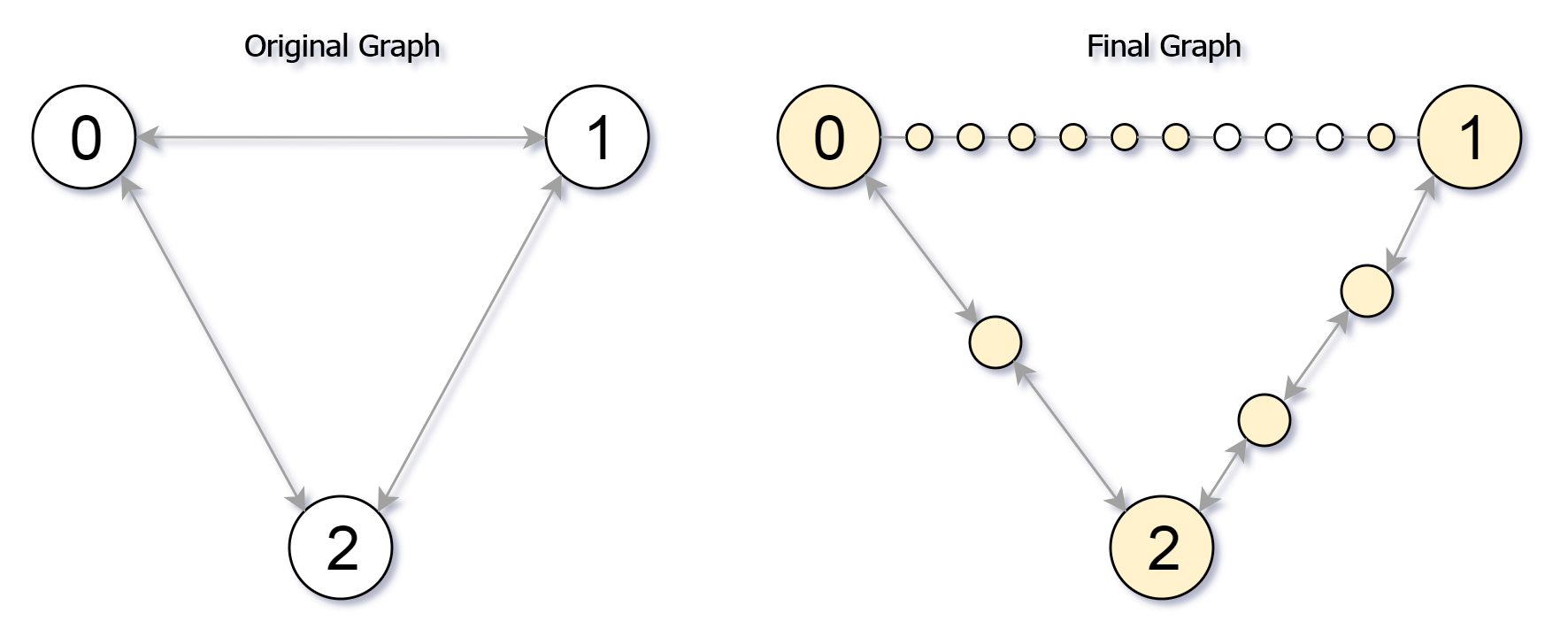Example 2:

Input: edges = [[0,1,4],[1,2,6],[0,2,8],[1,3,1]], M = 10, N = 4
Output: 23

Note:

1. 0 <= edges.length <= 10000
2. 0 <= edges[i] < edges[i] < N
3. There does not exist any i != j for which edges[i] == edges[j] and edges[i] == edges[j].
4. The original graph has no parallel edges.
5. 0 <= edges[i] <= 10000
6. 0 <= M <= 10^9
7. 1 <= N <= 3000

# Solution: Dijkstra Shortest Path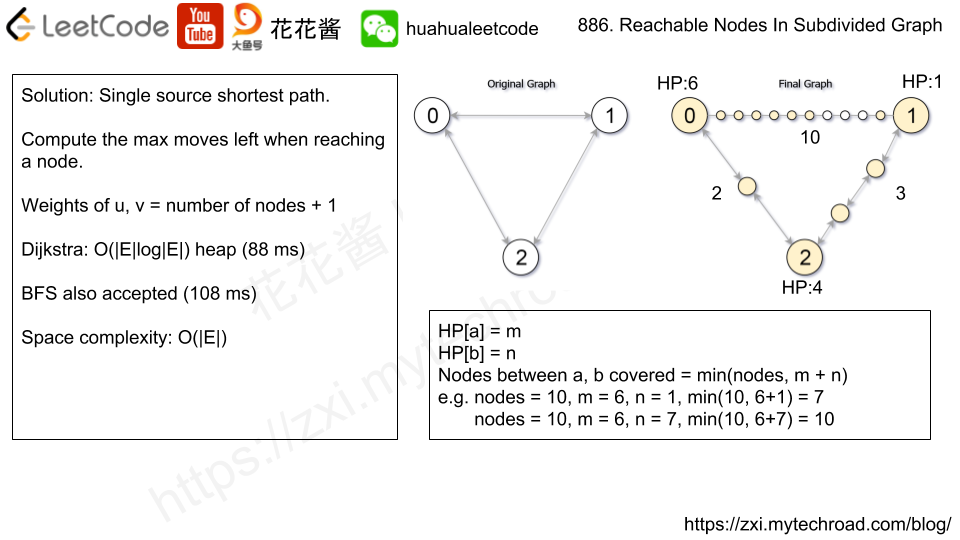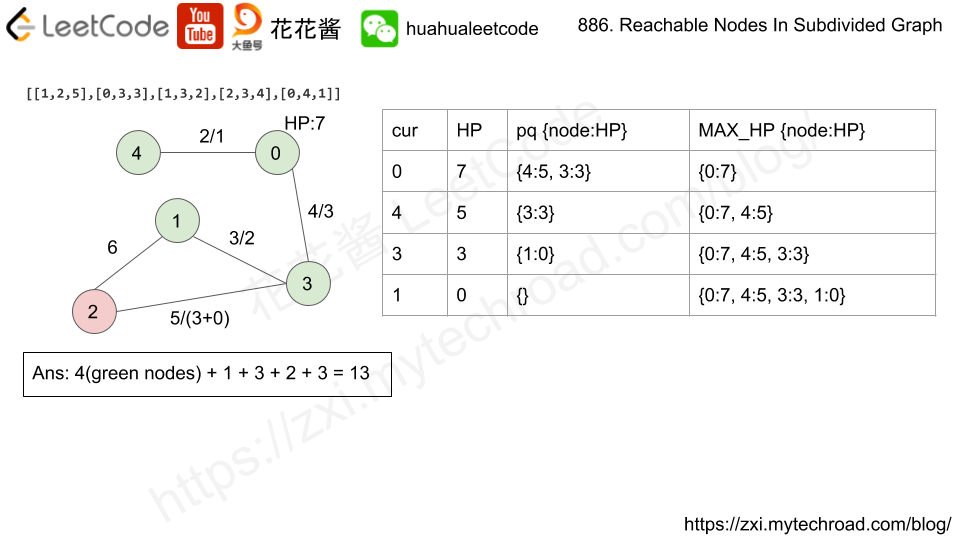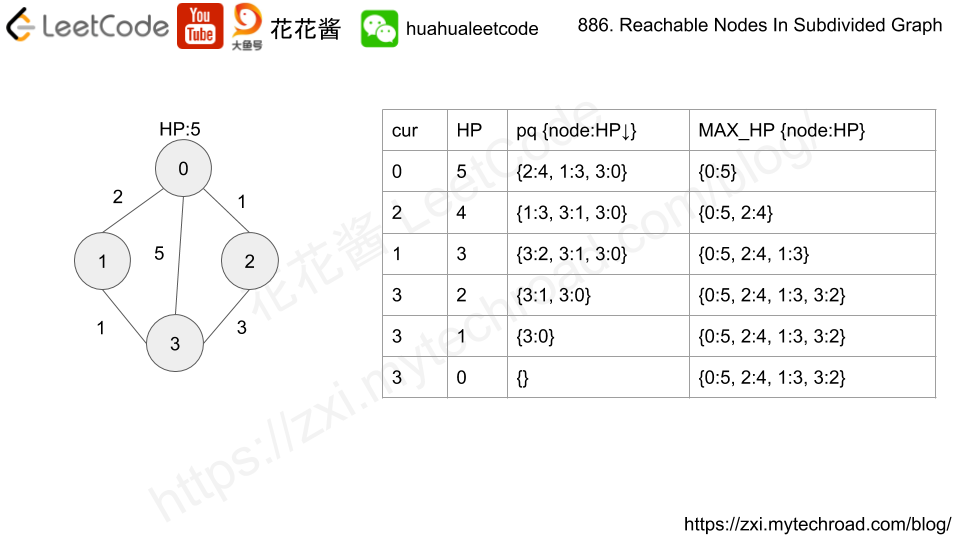Compute the shortest from 0 to rest of the nodes. Use HP to mark the maximum moves left to reach each node.

HP[u] = a, HP[v] = b, new_nodes[u][v] = c

nodes covered between a<->b = min(c, a + b)

Time complexity: O(ElogE)

Space complexity: O(E)

C++

Optimized Dijkstra (replace hashmap with vector)

Using SPFA

BFS

# Problem

Suppose LeetCode will start its IPO soon. In order to sell a good price of its shares to Venture Capital, LeetCode would like to work on some projects to increase its capital before the IPO. Since it has limited resources, it can only finish at most k distinct projects before the IPO. Help LeetCode design the best way to maximize its total capital after finishing at most k distinct projects.

You are given several projects. For each project i, it has a pure profit Pi and a minimum capital of Ci is needed to start the corresponding project. Initially, you have W capital. When you finish a project, you will obtain its pure profit and the profit will be added to your total capital.

To sum up, pick a list of at most k distinct projects from given projects to maximize your final capital, and output your final maximized capital.

Example 1:

Input: k=2, W=0, Profits=[1,2,3], Capital=[0,1,1].

Output: 4

Explanation: Since your initial capital is 0, you can only start the project indexed 0.
After finishing it you will obtain profit 1 and your capital becomes 1.
With capital 1, you can either start the project indexed 1 or the project indexed 2.
Since you can choose at most 2 projects, you need to finish the project indexed 2 to get the maximum capital.
Therefore, output the final maximized capital, which is 0 + 1 + 3 = 4.


Note:

1. You may assume all numbers in the input are non-negative integers.
2. The length of Profits array and Capital array will not exceed 50,000.
3. The answer is guaranteed to fit in a 32-bit signed integer.

# Solution: Greedy

For each round, find the most profitable job whose capital requirement <= W.

Finish that job and increase W.

Brute force (TLE)

Time complexity: O(kn)

Space complexity: O(1)

C++

Use priority queue and multiset to track doable and undoable projects at given W.

Time complexity: O(nlogn)

Space complexity: O(n)

Or use an array and sort by capital

# Problem

Given several boxes with different colors represented by different positive numbers.
You may experience several rounds to remove boxes until there is no box left. Each time you can choose some continuous boxes with the same color (composed of k boxes, k >= 1), remove them and get k*k points.
Find the maximum points you can get.

Example 1:
Input:

Output:

Explanation:

[1, 3, 2, 2, 2, 3, 4, 3, 1]
----> [1, 3, 3, 4, 3, 1] (3*3=9 points)
----> [1, 3, 3, 3, 1] (1*1=1 points)
----> [1, 1] (3*3=9 points)
----> [] (2*2=4 points)


Note: The number of boxes n would not exceed 100.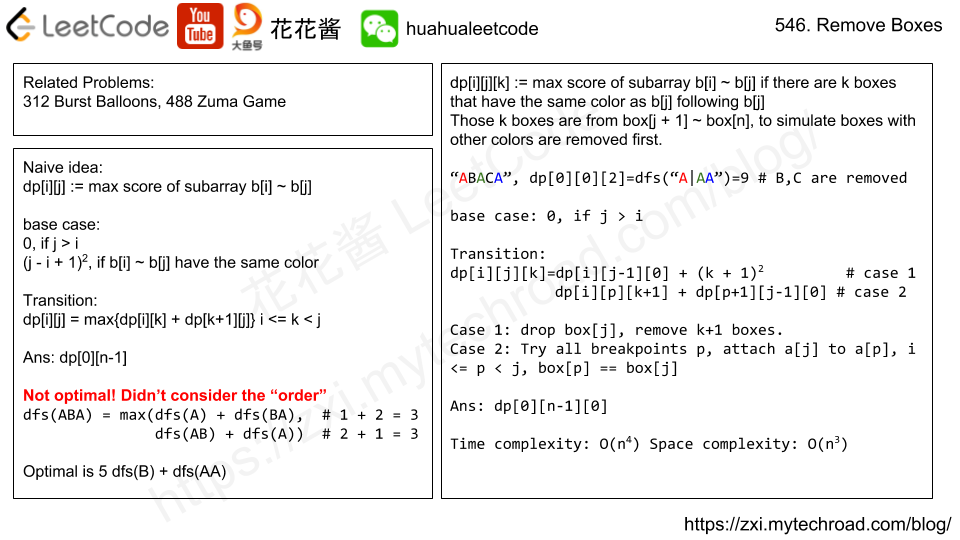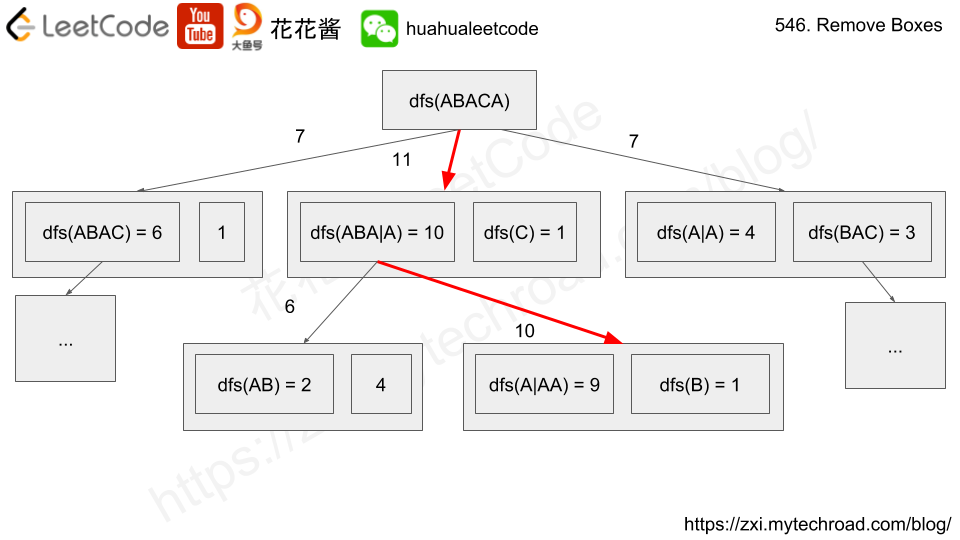# Solution: Recursion + Memorization

Use dp[l][r][k] to denote the max score of subarray box[l] ~ box[r] with k boxes after box[r] that have the same color as box[r]

box[l], box[l+1], …, box[r], box[r+1], …, box[r+k]

e.g. “CDABACAAAAA”

dp is the max score of [ABACA] followed by [AAAA]
dp is the max score of [ABACA] followed by [AAA]

base case: l > r, empty array, return 0.
Transition:
dp[l][r][k] = max(dp[l][r-1] + (k + 1)*(k + 1),  # case 1
dp[l][i][k+1] + dp[i+1][r-1])  # case 2
# "ABACA|AAAA"
# case 1: dp("ABAC") + score("AAAAA") drop j and the tail.
# case 2: box[i] == box[r], l <= i < r, try all break points
# max({dp("A|AAAAA") + dp("BAC")}, {dp("ABA|AAAAA") + dp("C")})

Time complexity: O(n^4)

Space complexity: O(n^3)

C++

Optimized

Use a HashTable to replace the 3D DP array since the DP array could be sparse when many consecutive boxes are the same color.

# Problem

There are G people in a gang, and a list of various crimes they could commit.

The i-th crime generates a profit[i] and requires group[i] gang members to participate.

If a gang member participates in one crime, that member can’t participate in another crime.

Let’s call a profitable scheme any subset of these crimes that generates at least P profit, and the total number of gang members participating in that subset of crimes is at most G.

How many schemes can be chosen?  Since the answer may be very large, return it modulo 10^9 + 7.

Example 1:

Input: G = 5, P = 3, group = [2,2], profit = [2,3]
Output: 2
Explanation:
To make a profit of at least 3, the gang could either commit crimes 0 and 1, or just crime 1.
In total, there are 2 schemes.


Example 2:

Input: G = 10, P = 5, group = [2,3,5], profit = [6,7,8]
Output: 7
Explanation:
To make a profit of at least 5, the gang could commit any crimes, as long as they commit one.
There are 7 possible schemes: (0), (1), (2), (0,1), (0,2), (1,2), and (0,1,2).


Note:

1. 1 <= G <= 100
2. 0 <= P <= 100
3. 1 <= group[i] <= 100
4. 0 <= profit[i] <= 100
5. 1 <= group.length = profit.length <= 100

# Solution: DP

Time complexity: O(KPG)

Space complexity: O(KPG)

C++

Space complexity: O(PG)

v1: Dimension reduction by copying.

v2: Dimension reduction by using rolling array.

Mission News Theme by Compete Themes.